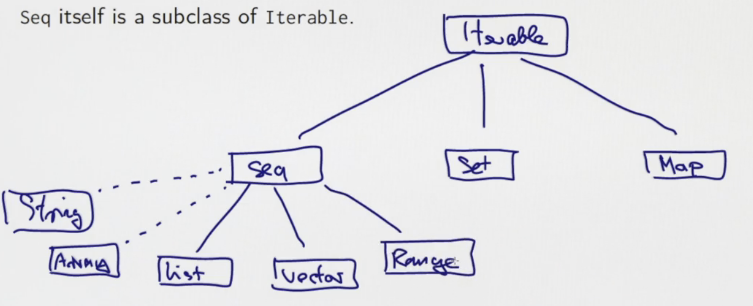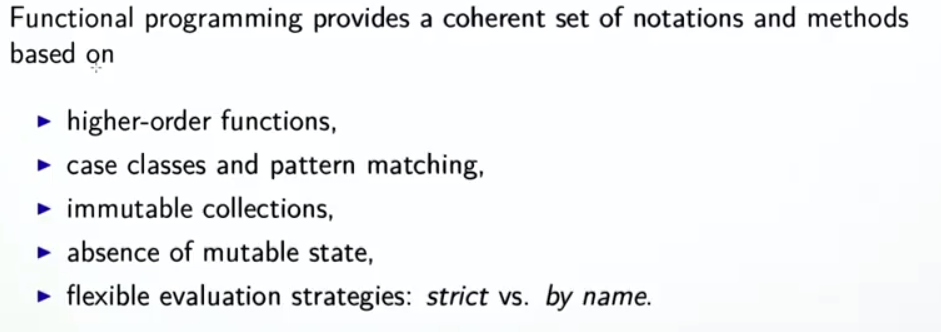[TOC]

# 6.1 - Other Collections

so far: only seen List. → more (immutable) collections.

### vector

List: is linear -- access to head is faster than middle or end element. Vector: better rand access performance.

represented as very shallow trees(32-split at each node)`Vector` support similar operations as List (head, tail,map, fold) , except concat `::`, instead Vectors have `+:` and `:+`:

• `x +: xs` create new vector with x in head
• `xs :+ x` create new vector with x in tail

implementation of `:+`: create copies### Seq

`Seq` is base class for `Vector` and `List`, `Seq` itself is subclass of `Iterable`.

Hierarchy of Iterables:### Array ans String

Array and String support same op as Seq, can implicitly be converted to seq when needed. But they are NOT subclasses of Seq as they come from java.

```scala> val xs: Array[Int] = Array(1,2,3)
xs: Array[Int] = Array(1, 2, 3)

scala> xs map (2*_)
res3: Array[Int] = Array(2, 4, 6)

scala> val ys = "Hello"
ys: String = Hello

scala> ys filter (_.isUpper)
res4: String = H
```

### Range

represents a seq of evenly spaced integers.

represented as an obj with 3 values: lower bound, upper bound, step value.

3 op:

• `to`: inclusive
• `until`: exclusive
• `by`: step value

1 to 6 // 1 2 3 4 5 6 1 until 6 // 1 2 3 4 5 1 to 6 by 2 // 1 3 5 6 to 1 by -2 // 6 4 2

### More ops on Seq

• `xs exists p`
• `xs forall p`
• `xs zip ys`: takes 2 seq, returns a single seq, each elem is a pair

scala> List(1,2,3) zip Vector(2,3,4) res7: List[(Int, Int)] = List((1,2), (2,3), (3,4))

• `xs.unzip`: reverse zip, return 2 lists

• `xs flatMap f`: apply f to all elements, and concat the results

scala> "hello" flatMap (c => List('.', c)) res9: String = .h.e.l.l.o

• `flatten`: flatten a seq of seq into just one seq...

`xs flatMap f = (xs map f).flatten`

The result is concated, instead of being a list of list.

• `xs.sum`/`xs.product`: for numeric collection
• `xs.max`/`xs.min`: an ordering must exist

### Examples

• cartesen product: 1..M x 1..N:

`(1 to M) flatMap( x => (1 to N) map (y => (x,y)) )`

• scalar of 2 vectors

def scalaProd(xs: Vector[Double], ys: Vector[Double]): Double = (xs zip ys) map (xy => xy._1 * xy._2).sum

alternative: use pattern matching in map (need to add braces `{}`!):

```(xs zip ys) map ( {case(x,y) => x*y}).sum
```
• test isPrime for a number

`def isPrime(n: Int): Boolean = (2 until n) forall (d => n%d!=0)`

# 6.2 - Combinatorial Search and For-Expressions

goal: handle nested seq.

⇒ extend usage of higher order functions instead of using nested loops.

example:

given n, find all (i,j) st: 1<=j<i<=n, and i+j is prime.

(1 until n) flatMap ( i => (1 until i) map (j => (i,j)) ) filter ( {case(i,j) => isPrime(i+j)})

this works, but less understandable... ⇒ for expressions.

### for/yield expressions

example: class of person

`case class Person(name: String, age: Int)`

To obtain names of persons over 20 years old:

`persons filter (_.age>20) map (_.name)`

equivalent to :

`for (p <- person if p.age > 20) yield p.name`

The for expression is similar to jave for-loop, except it builds a list of the results of all iterations.

for expression form:

`for( s ) yield e` where `s` can contain generators and filters:

• generator: `p<-e` where `e` is a collection, `p` is a pattern
• filter: `if f` where t is boolean expr

rewrite the prime sum example:

```for{
i <- 1 until n
j <- 1 until i
if isPrime(i+j)
} yield (i,j)
```

exercice: write the scalaProd using for expr:

```def scalaProd(xs: Vector[Double], ys: Vector[Double]): Double =
(for( (x,y) <- xs zip ys ) yield x*y ) sum
```

more on scala for expr: http://www.artima.com/pins1ed/for-expressions-revisited.html

# 6.3 - Combinatorial Search Example

goal: combine `set` and `for`-expr to solve the n-queens problem.

### Sets

3 fundamental iterable collection types: seq, set, map.

• set support most operations on seq (c.f. doc on Iterable)
• sets are unordered
• set don't have dup elements
• contains: `s contains e`

### Example: N-Queens

8*8 chess board, 8 queens ⇒ places queens st: no two queen in same row/col/diag

algo: put one queen in each row (in the right column)

recursive solution: suppose already have solutions for first n-1 rows. represent each (partial)solution as a list of column index.

return all possible solutions (as a Set).

```def queens(n: Int): Set[List[Int]] = {
def placeQueens(k: Int): Set[List[Int]] = ???// place first k rows
placeQueens(n)
}
```

Fill in the helper function:

```def placeQueens(k: Int): Set[List[Int]] = {
if (k==0) Set(List())
else for{
queens <- placeQueens(k-1) // queens(List[Int]) = one solution to k-1
c <- 0 until n
if isSafe(c, queens) // if column c doesn't conflict with the partial solution queens
} yield c::queens // the kth row solution is in head of list
}
```

Now fill in the function isSafe that check if a column is valid wrt queens for above rows:

```def isSafe(col: Int, queens: List[Int]): Boolean = {
val k = queens.length // current row is k
val indexedQueens = (k-1 to 0 by -1) zip queens // List[(row, col)]
indexedQueens forall {
case(r,c) => c!=col && k-r!=math.abs(c-col) }
}
```

Now the queens function will work.

Write another function to print the solution out:

```def show(queens: List[Int]): String = {
val lines = for (col <- queens.reverse)
yield Vector.fill(queens.length("* ").updated(col, "X ").mkString
"\n" + lines.mkString("\n")
}
(queens(4) map show) mkString "====\n"
```

# 6.4 - Maps

`Map[Key, Value]`

ex:

```val romanNumerals = Map("I" ->1, "V" ->5, "X" -> 10)
val captial = Map("France" -> "Paris", "US" -> "Washington")
```

Maps are iterables and functions: maps Key to Value like a function call

ex. `romanNumerals("I")`

will throw `NoSuchElementException` if the key is not in map.

⇒ use `get`:

```scala> captial("France")
res3: String = Paris

scala> captial get "France"
res4: Option[String] = Some(Paris)

scala> captial get "China"
res5: Option[String] = None
```

have a look at the Option type:

### The Option Type

definition:

```trait Option[+A]
case class Some[+A](value: A] extends Option[A]
object None extends Option[Nothing]
```

so the get function of map gives:

• `None` if key is not in map
• `Some(x)` if map associates key to x

since `Some` is case class, can use pattern matching to decompose.

```def showCaptical(country: String) = captial.get(country) match{
case Some(cap) => cap
case None => "missing data"
}
```

### Sorted and GroupBy

two useful SQL queries.

• orderBy can be expressed using sortWith and sorted:

val fruit = List("apple", "pear", "orange", "pineapple") scala> fruit sortWith (.length < .length) res7: List[String] = List(pear, apple, orange, pineapple) scala> fruit.sorted res8: List[String] = List(apple, orange, pear, pineapple)

• `GroupBy(f)`: partition a collection into a map of collections according to `f`

scala> fruit groupBy (_.head) res9: scala.collection.immutable.Map[Char,List[String]] = Map(p -> List(pear, pineapple), a -> List(apple), o -> List(orange))

### map Example: polynomials

can be seen as map from exponents to coefficients:⇒ represent polynm as maps

```class Poly(val terms: Map[Int, Double]) {
def + (other: Poly) = new Poly(terms ++ // ++ on maps: will override older entries in terms!
(other.terms maps adjust)) // adjust defined below

def adjust(term: (Int, Double)):(Int, Double) = {
val (exp, coeff) = term
terms get exp match{
case None => Pair(exp, coeff) // or write: exp -> coeff
case Some(coeff1) => Pair(exp, coeff+coeff1)
}
}
override def toString =
(for( (exp, coeff) <- terms.toList.sorted.reverse) yield coeff+"x^"+exp) mkString "+ "
}
```

### default values

`withDefulatValue`: makes a map into a total map

rewrite the poly example:

```class Poly(terms0: Map[Int, Double]) {
val terms = terms0 withDefaultValue 0.0
def + (other: Poly) = new Poly(terms ++ // ++ on maps: will override older entries in terms!
(other.terms maps adjust)) // adjust defined below

def adjust(term: (Int, Double)):(Int, Double) = {
val (exp, coeff) = term
exp -> (coeff + terms(exp))
}
override def toString =
(for( (exp, coeff) <- terms.toList.sorted.reverse) yield coeff+"x^"+exp) mkString "+ "
}
}
```

change to variable parameter for better constructor calling: add another constructor

```def this(bindings: (int, Double)*) = // bindings is s sequence
this(bindings.toMap)
```

Exercie: re-implement the `+` using foldLeft :

```def + (other: Poly) =
new Poly( (other.terms foldLeft terms)(addTerm) )
def addTerm(terms:Map[Int, Double], term: (Int, Double)): Map[Int, Double] = {
val (exp, coeff) = term
terms + ( exp -> (coeff + terms(exp)) ) // map + (k,v) updates the map
}
```

# 6.5 - Putting the Pieces Together: T9 keyboard mnem

convert a seq of numbers to a scentence

```val mnem  = Map('2'->"ABC", '3'->"DEF", 4->"GHI", '5'->"JKL",
'6'->"MNO", '7'->"PQRS", '8'->"TUV", '9'->"WXYZ" )
val in = Source.fromURL("...")
val words = in.getLines.toList // dictionary: list of valid words
```

goal: design a `translate(phoneNumber)` that produces all phrases of words that can serve as mnemonics(助忆) for a phone number.

Step 1: invert the mnem map to a map from A~Z to 2~9:

```val charCode: Map[Char, Char] =
for ( (digit, str) <-mnem; ltr<-str) yield ltr->digit
```

Step 2: map a word to the string it represents, ex. "java"->"5282"

```def wordCode(word: String):String =
word.toUpperCase map charCode
```

Step 3: maps from digit string to all possible valid strings, ex. "5282"->List("java", "kata",...) ⇒ just a groupby!!

```val wordsForNum: Map[String, Seq[String]] =
words groupBy wordCode
```

→ Error: key not found: "-", some words contains a "-"... → drop such words...

`val words = in.getLines.toList filter (wd => wd forall (ch => ch.isLetter))`

Step 4: return all ways to encode a number as a list of words → recursive, recurse on the nb of first words~

```def encode(number: String): Set[List[String]] = {
if(number.isEmpty) Set(List())
else{
val phraseList = for{
split <- 1 to number.length // the first word in phrase uses numbers[1:split]
firstWd <- wordsForNum[ number.take(split) ]
followingWds <- encode(number.drop(split))
} yield firstWd::follwingWds
phraseList.toSet
}
}
```

⇒ error in the line with `wordsForNum`: key not found... ⇒ `wordsForNum["7"]` will give error ⇒ make the `wordsForNum` a total map, using withDefaultValue

```val wordsForNum: Map[String, Seq[String]] =
(words groupBy wordCode) withDefaultValue( Seq() )
```

Step 5: return strings (instead of List[String])

```def translate(number: String): Set[String] = {
encode(number) map (_.mkString(" "))
}
```

summary:# Conclusionhttps://www.scala-exercises.org/

#### Part 7 of series «Functional Programming Principles in Scala»：

comments powered by Disqus